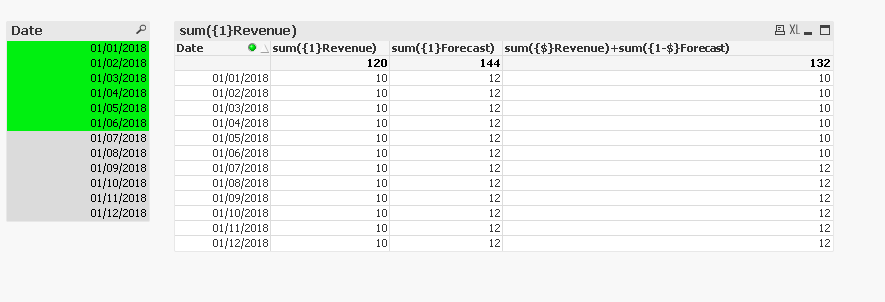Announcements
The way to achieve your own success is the willingness to help somebody else. Go for it!
cancel
Showing results for
Did you mean:Contributor III

## Set analysis and user selections

I want to create a chart spanning the 12 months of the year.

Based on what month(s)the user has selected in the filters.

So if the user has selected the months Jan-April, i would like an expression in the chart that does the following:

If month is Jan to April  i would like a set analysis to show the sum of Revenue

If the month is May to Dec i would like a set analysis to show the sum of Forecast

I have created variables that calculate these values, vRev and vFcast

Is this possible?

Thank you

4 RepliesMVP
I believe it is possibleSpecialist II

Hi,

I think you need to share a bit more about the model you have.

So I can create a simple model where Revenue & Forecast are fields (not variables) in a table with the date & then use set analysis to get the kind of behaviour you want;But depending on your model this may or may not be a helpful approach.

Cheers,

Chris.Contributor III
Author

Hi Chris,

I have this expression that works as I want it to.  It calculates actual and forecasted sales quantities based on the users selection of dates in the filters.  it works because _volume_total and quantity are fields.

sum( { <year={"=\$(=max(year))"}, month={"<=\$(=max(month))"}>}_volume_total)
+
sum( {<year={"\$(=max(year))"}, month={">\$(=max(month))"}>}quantity)

The problem is in the model there is no field for sales revenue.  It is a combination of many fields to display or calculate it a variable is used (that includes its own set analysis)

The variable vREV represents revenue and looks like

Sum(\$(vRevBase))
+
Sum(\$(vDiscComp))
+
Sum({\$<ROCKType={'Aggregates'}>}\$(vEnviroFees))
+
Sum({\$<ROCKType={'Aggregates'}>}\$(vGovtFees))
+
Sum(\$(vDiscPayment))
+
Sum(\$(vCreditDebit))
-
Sum(\$(vRebate))

I can't recreate the set analysis at the top that uses _volume_total or quantity with this variable - it never works...

Here is what i try to do:  the \$(vREV) causes it to fail.  revenue is just a field and this part works fine

sum( { <year={"=\$(=max(year))"}, month={"<=\$(=max(month))"}>}\$(vREV))
+
sum( {<year={"\$(=max(year))"}, month={">\$(=max(month))"}>}revenue)Specialist II

That does all look complex. My immediate thought is that if you can you should try to build into the scripting something that at least gets you closer, then the simple set analysis should work.

If you cannot do that I think you are going to need to unpack your variables somewhat. There is a requirement to use aggr between two aggregations, so sum(sum(x)) needs to be sum(aggr(sum())) & other complexities in terms of how/when the \$() expansions are done, that to be honest I should probably understand more than I do. You would need to think that through to do the scripting approach anyway.

Looking at vRev I assume vRevBase etc also variables - are they similarly complex? Post up a bit more detail & I can take a look on Monday.

Cheers,

Chris.Community Browser